# Triplet STDP synapse tutorial

In this tutorial, we will learn to formulate triplet rule (which considers sets of three spikes, i.e., two presynaptic and one postsynaptic spikes or two postsynaptic and one presynaptic spikes) for Spike Timing-Dependent Plasticity (STDP) learning model using NESTML and simulate it with NEST simulator.

:

%matplotlib inline
import matplotlib.pyplot as plt
import nest
import numpy as np
import os
import re

from pynestml.codegeneration.nest_code_generator_utils import NESTCodeGeneratorUtils

np.set_printoptions(suppress=True)


Early experiments in Bi and Poo (1998)  have shown that a sequence of $$n$$ pairs of “pre then post” spikes result in synaptic potentiation and $$n$$ pairs of “post then pre” result in synaptic depression. Later experiments have shown that these pairs of spikes do not necessarily describe the synaptic plasiticity behavior. Other variables like calcium concentration or postsynaptic membrane potential play an important role in potentiation or depression.

Experiments conducted by Wang et al.,  and Sjöström et al.,  using triplet and quadruplets of spikes show that the classical spike-dependent synaptic plasticity alone cannot explain the results of the experiments. The triplet STDP model formulated by Pfister and Gerstner in  assume that a combination of pairs and triplets of spikes triggers the synaptic plasticity and thus reproduce the experimental results.

## Triplet STDP model

The synaptic transmission is formulated by a set of four differential equations as defined below.

\begin{equation} \frac{dr_1}{dt} = -\frac{r_1(t)}{\tau_+} \quad \text{if } t = t^{pre}, \text{ then } r_1 \rightarrow r_1+1 \end{equation}

\begin{equation} \frac{dr_2}{dt} = -\frac{r_2(t)}{\tau_x} \quad \text{if } t = t^{pre}, \text{ then } r_2 \rightarrow r_2+1 \end{equation}

\begin{equation} \frac{do_1}{dt} = -\frac{o_1(t)}{\tau_-} \quad \text{if } t = t^{post}, \text{ then } o_1 \rightarrow o_1+1 \end{equation}

\begin{equation} \frac{do_2}{dt} = -\frac{o_2(t)}{\tau_y} \quad \text{if } t = t^{post}, \text{ then } o_2 \rightarrow o_2+1 \end{equation}

Here, $$r_1$$ and $$r_2$$ are the trace variables that detect the presynaptic events when a spike occurs at the presynaptic neuron at time $$t^{pre}$$. These variables increase when a presynaptic spike occurs and decrease back to 0 otherwise with time constants $$\tau_+$$ and $$\tau_x$$ respectively. Similarly, the variables $$o_1$$ and $$o_2$$ denote the trace variables that detect the postsynaptic events at a spike time $$t^{post}$$. In the absence of a postsynaptic spike, the vairables decrease their value with a time constant $$\tau_-$$ and $$\tau_y$$ respectively. Presynaptic variables, for example, can be the amount of glutamate bound and the postsynaptic receptors can determine the influx of calcium concentration through voltage-gated $$Ca^{2+}$$ channels.

The weight change in the model is formulated as a function of the four trace variables defined above. The weight of the synapse decreases after the presynaptic spikes arrives at $$t^{pre}$$ by an amount proportional to the postsynaptic variable $$o_1$$ but also depends on the second presynaptic variable $$r_2$$.

\begin{equation} w(t) \rightarrow w(t) - o_1(t)[A_2^- + A_3^- r_2(t - \epsilon)] \quad \text{if } t = t^{pre} \end{equation}

Similarly, a postsynaptic spike at time $$t^{post}$$ increases the weight of the synapse and is dependent on the presynaptic variable $$r_1$$ and the postsynaptic variable $$o_2$$.

\begin{equation} w(t) \rightarrow w(t) + r_1(t)[A_2^+ + A_3^+ o_2(t - \epsilon)] \quad \text{if } t = t^{post} \end{equation}

Here, $$A_2^+$$ and $$A_2^-$$ denote the amplitude of the weight change whenever there is a pre-post pair or a post-pre pair. Similarly, $$A_3^+$$ and $$A_3^-$$ denote the amplitude of the triplet term for potentiation and depression, respectively.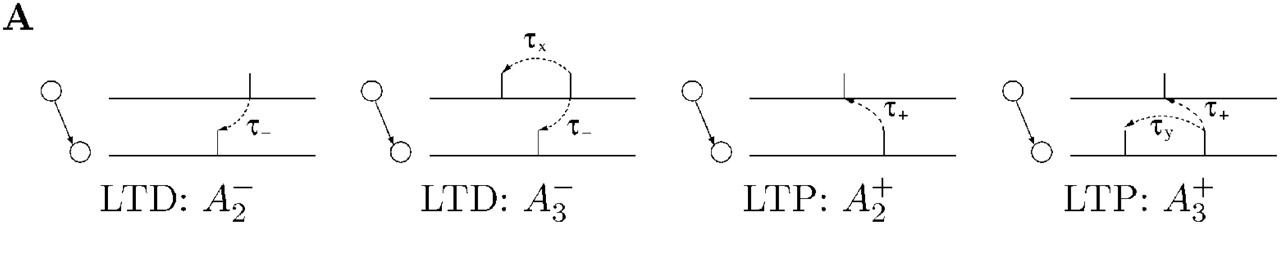## Generating code with NESTML

### Triplet STDP model in NESTML

In this tutorial, we will use a very simple integrate-and-fire model, where arriving spikes cause an instantaneous increment of the membrane potential, the “iaf_psc_delta” model. It is already provided with NESTML in the models/neurons directory.

We will be formulating two types of interactions between the spikes, namely All-to-All interaction and Nearest-spike interaction.

$$\textit{All-to-All Interation}$$ - Each postsynaptic spike interacts with all previous postsynaptic spikes and vice versa. All the internal variables $$r_1, r_2, o_1,$$ and $$o_2$$ accumulate over several postsynaptic spike timimgs.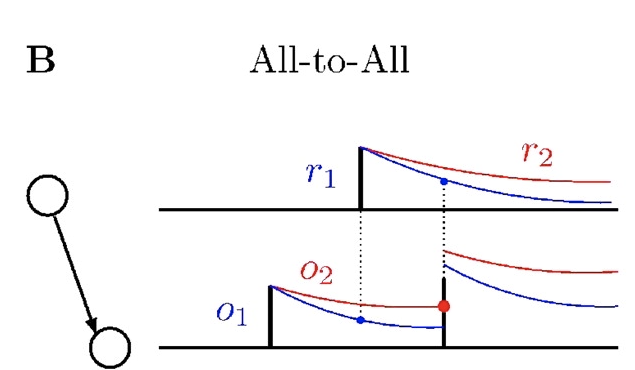:

nestml_triplet_stdp_model = '''
synapse stdp_triplet:

state:
w nS = 1 nS

tr_r1 real = 0.
tr_r2 real = 0.
tr_o1 real = 0.
tr_o2 real = 0.

parameters:
d ms = 1 ms  @nest::delay

tau_plus ms = 16.8 ms  # time constant for tr_r1
tau_x ms = 101 ms  # time constant for tr_r2
tau_minus ms = 33.7 ms  # time constant for tr_o1
tau_y ms = 125 ms  # time constant for tr_o2

A2_plus real = 7.5e-10
A3_plus real = 9.3e-3
A2_minus real = 7e-3
A3_minus real = 2.3e-4

Wmax nS = 100 nS
Wmin nS = 0 nS

equations:
tr_r1' = -tr_r1 / tau_plus
tr_r2' = -tr_r2 / tau_x
tr_o1' = -tr_o1 / tau_minus
tr_o2' = -tr_o2 / tau_y

input:
pre_spikes <- spike
post_spikes <- spike

output:
spike

# increment post trace values
tr_o1 += 1
tr_o2 += 1

# potentiate synapse
w_ nS = w + tr_r1 * ( A2_plus + A3_plus * tr_o2 )
w = min(Wmax, w_)

# increment pre trace values
tr_r1 += 1
tr_r2 += 1

# depress synapse
w_ nS = w  -  tr_o1 * ( A2_minus + A3_minus * tr_r2 )
w = max(Wmin, w_)

# deliver spike to postsynaptic partner
deliver_spike(w, d)
'''

:

# Generate code for All-to-All spike interaction
module_name, neuron_model_name, synapse_model_name = NESTCodeGeneratorUtils.generate_code_for("../../../models/neurons/iaf_psc_delta.nestml",
nestml_triplet_stdp_model,
post_ports=["post_spikes"])
nest.Install(module_name)

[7,iaf_psc_deltaf626ae1d8042411a8b394014f08dca4a_nestml, WARNING, [59:8;60:8]]: Variable 'G' has the same name as a physical unit!
[8,iaf_psc_deltaf626ae1d8042411a8b394014f08dca4a_nestml, WARNING, [77:8;77:26]]: Variable 'h' has the same name as a physical unit!
[14,stdp_tripletf626ae1d8042411a8b394014f08dca4a_nestml, WARNING, [13:8;13:28]]: Variable 'd' has the same name as a physical unit!
[17,stdp_tripletf626ae1d8042411a8b394014f08dca4a_nestml, WARNING, [47:16;47:16]]: Implicit casting from (compatible) type 'nS' to 'real'.
[20,stdp_tripletf626ae1d8042411a8b394014f08dca4a_nestml, WARNING, [56:16;56:16]]: Implicit casting from (compatible) type 'nS' to 'real'.
[27,iaf_psc_deltaf626ae1d8042411a8b394014f08dca4a_nestml, WARNING, [59:8;60:8]]: Variable 'G' has the same name as a physical unit!
[28,iaf_psc_deltaf626ae1d8042411a8b394014f08dca4a_nestml, WARNING, [77:8;77:26]]: Variable 'h' has the same name as a physical unit!
[31,stdp_tripletf626ae1d8042411a8b394014f08dca4a_nestml, WARNING, [13:8;13:28]]: Variable 'd' has the same name as a physical unit!
[61,iaf_psc_deltaf626ae1d8042411a8b394014f08dca4a_nestml__with_stdp_tripletf626ae1d8042411a8b394014f08dca4a_nestml, WARNING, [59:8;60:8]]: Variable 'G' has the same name as a physical unit!
[62,iaf_psc_deltaf626ae1d8042411a8b394014f08dca4a_nestml__with_stdp_tripletf626ae1d8042411a8b394014f08dca4a_nestml, WARNING, [77:8;77:26]]: Variable 'h' has the same name as a physical unit!
[67,stdp_tripletf626ae1d8042411a8b394014f08dca4a_nestml__with_iaf_psc_deltaf626ae1d8042411a8b394014f08dca4a_nestml, WARNING, [13:8;13:28]]: Variable 'd' has the same name as a physical unit!
[68,stdp_tripletf626ae1d8042411a8b394014f08dca4a_nestml__with_iaf_psc_deltaf626ae1d8042411a8b394014f08dca4a_nestml, WARNING, [47:16;47:16]]: Implicit casting from (compatible) type 'nS' to 'real'.
[71,stdp_tripletf626ae1d8042411a8b394014f08dca4a_nestml__with_iaf_psc_deltaf626ae1d8042411a8b394014f08dca4a_nestml, WARNING, [56:16;56:16]]: Implicit casting from (compatible) type 'nS' to 'real'.
[76,iaf_psc_deltaf626ae1d8042411a8b394014f08dca4a_nestml, WARNING, [77:8;77:26]]: Variable 'h' has the same name as a physical unit!
[80,iaf_psc_deltaf626ae1d8042411a8b394014f08dca4a_nestml__with_stdp_tripletf626ae1d8042411a8b394014f08dca4a_nestml, WARNING, [77:8;77:26]]: Variable 'h' has the same name as a physical unit!
[85,stdp_tripletf626ae1d8042411a8b394014f08dca4a_nestml__with_iaf_psc_deltaf626ae1d8042411a8b394014f08dca4a_nestml, WARNING, [13:8;13:28]]: Variable 'd' has the same name as a physical unit!
[90,stdp_tripletf626ae1d8042411a8b394014f08dca4a_nestml__with_iaf_psc_deltaf626ae1d8042411a8b394014f08dca4a_nestml, WARNING, [13:8;13:28]]: Variable 'd' has the same name as a physical unit!


$$\textit{Nearest spike Interation}$$ - Each postsynaptic spike interacts with only the last spike. Hence no accumulation of the trace variables occurs. The variables saturate at 1. This is achieved by updating the variables to the value of 1 instead of updating by at step of 1. In this way, the synapse forgets all other previous spikes and keeps only the memory of the last one.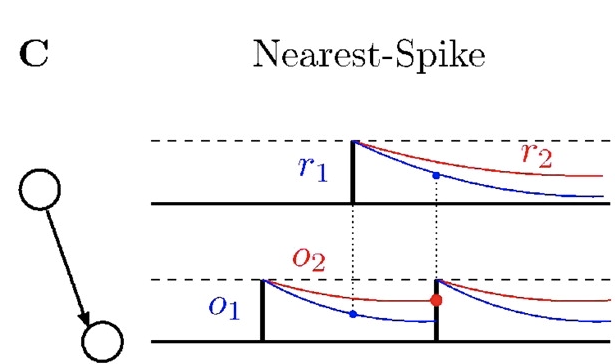:

nestml_triplet_stdp_nn_model = '''
synapse stdp_triplet_nn:

state:
w nS = 1 nS

tr_r1 real = 0.
tr_r2 real = 0.
tr_o1 real = 0.
tr_o2 real = 0.

parameters:
d ms = 1 ms  @nest::delay

tau_plus ms = 16.8 ms  # time constant for tr_r1
tau_x ms = 101 ms  # time constant for tr_r2
tau_minus ms = 33.7 ms  # time constant for tr_o1
tau_y ms = 125 ms  # time constant for tr_o2

A2_plus real = 7.5e-10
A3_plus real = 9.3e-3
A2_minus real = 7e-3
A3_minus real = 2.3e-4

Wmax nS = 100 nS
Wmin nS = 0 nS

equations:
tr_r1' = -tr_r1 / tau_plus
tr_r2' = -tr_r2 / tau_x
tr_o1' = -tr_o1 / tau_minus
tr_o2' = -tr_o2 / tau_y

input:
pre_spikes <- spike
post_spikes <- spike

output:
spike

# increment post trace values
tr_o1 = 1
tr_o2 = 1

# potentiate synapse
#w_ nS = Wmax * ( w / Wmax + tr_r1 * ( A2_plus + A3_plus * tr_o2 ) )
w_ nS = w + tr_r1 * ( A2_plus + A3_plus * tr_o2 )
w = min(Wmax, w_)

# increment pre trace values
tr_r1 = 1
tr_r2 = 1

# depress synapse
#w_ nS = Wmax * ( w / Wmax  -  tr_o1 * ( A2_minus + A3_minus * tr_r2 ) )
w_ nS = w  -  tr_o1 * ( A2_minus + A3_minus * tr_r2 )
w = max(Wmin, w_)

# deliver spike to postsynaptic partner
deliver_spike(w, d)
'''

:

# Generate code for nearest spike interaction model
module_name, neuron_model_name_nn, synapse_model_name_nn = NESTCodeGeneratorUtils.generate_code_for("../../../models/neurons/iaf_psc_delta.nestml",
nestml_triplet_stdp_nn_model,
post_ports=["post_spikes"])
nest.Install(module_name)

[7,iaf_psc_delta07edd451bf704570a7deb433b776f7ab_nestml, WARNING, [59:8;60:8]]: Variable 'G' has the same name as a physical unit!
[8,iaf_psc_delta07edd451bf704570a7deb433b776f7ab_nestml, WARNING, [77:8;77:26]]: Variable 'h' has the same name as a physical unit!
[14,stdp_triplet_nn07edd451bf704570a7deb433b776f7ab_nestml, WARNING, [13:8;13:28]]: Variable 'd' has the same name as a physical unit!
[17,stdp_triplet_nn07edd451bf704570a7deb433b776f7ab_nestml, WARNING, [48:16;48:16]]: Implicit casting from (compatible) type 'nS' to 'real'.
[20,stdp_triplet_nn07edd451bf704570a7deb433b776f7ab_nestml, WARNING, [58:16;58:16]]: Implicit casting from (compatible) type 'nS' to 'real'.
[27,iaf_psc_delta07edd451bf704570a7deb433b776f7ab_nestml, WARNING, [59:8;60:8]]: Variable 'G' has the same name as a physical unit!
[28,iaf_psc_delta07edd451bf704570a7deb433b776f7ab_nestml, WARNING, [77:8;77:26]]: Variable 'h' has the same name as a physical unit!
[31,stdp_triplet_nn07edd451bf704570a7deb433b776f7ab_nestml, WARNING, [13:8;13:28]]: Variable 'd' has the same name as a physical unit!
[61,iaf_psc_delta07edd451bf704570a7deb433b776f7ab_nestml__with_stdp_triplet_nn07edd451bf704570a7deb433b776f7ab_nestml, WARNING, [59:8;60:8]]: Variable 'G' has the same name as a physical unit!
[62,iaf_psc_delta07edd451bf704570a7deb433b776f7ab_nestml__with_stdp_triplet_nn07edd451bf704570a7deb433b776f7ab_nestml, WARNING, [77:8;77:26]]: Variable 'h' has the same name as a physical unit!
[67,stdp_triplet_nn07edd451bf704570a7deb433b776f7ab_nestml__with_iaf_psc_delta07edd451bf704570a7deb433b776f7ab_nestml, WARNING, [13:8;13:28]]: Variable 'd' has the same name as a physical unit!
[68,stdp_triplet_nn07edd451bf704570a7deb433b776f7ab_nestml__with_iaf_psc_delta07edd451bf704570a7deb433b776f7ab_nestml, WARNING, [48:16;48:16]]: Implicit casting from (compatible) type 'nS' to 'real'.
[71,stdp_triplet_nn07edd451bf704570a7deb433b776f7ab_nestml__with_iaf_psc_delta07edd451bf704570a7deb433b776f7ab_nestml, WARNING, [58:16;58:16]]: Implicit casting from (compatible) type 'nS' to 'real'.
[76,iaf_psc_delta07edd451bf704570a7deb433b776f7ab_nestml, WARNING, [77:8;77:26]]: Variable 'h' has the same name as a physical unit!
[80,iaf_psc_delta07edd451bf704570a7deb433b776f7ab_nestml__with_stdp_triplet_nn07edd451bf704570a7deb433b776f7ab_nestml, WARNING, [77:8;77:26]]: Variable 'h' has the same name as a physical unit!
[85,stdp_triplet_nn07edd451bf704570a7deb433b776f7ab_nestml__with_iaf_psc_delta07edd451bf704570a7deb433b776f7ab_nestml, WARNING, [13:8;13:28]]: Variable 'd' has the same name as a physical unit!
[90,stdp_triplet_nn07edd451bf704570a7deb433b776f7ab_nestml__with_iaf_psc_delta07edd451bf704570a7deb433b776f7ab_nestml, WARNING, [13:8;13:28]]: Variable 'd' has the same name as a physical unit!


## Results

### Spike pairing protocol

If we set the values of $$A_3^+$$ and $$A_3^-$$ to 0 in the above equations, the model becomes a classical STDP model. The authors in  tested this model to check if they could reproduce the experimental results of  and . They tested this with a pairing protocol which is a classic STDP protocol where the pairs of presynaptic and postsynaptic spikes shifted by $$\Delta{t}$$ are repeated at regular intervals of $$(1/\rho)$$. They showed that spike pairs repeated at high frequencies did not have considerable effect on the synaptic weights as shown by the experimental data.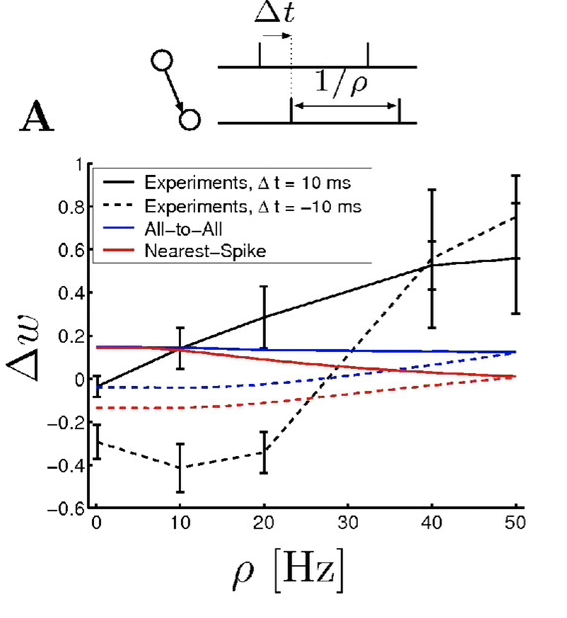### Triplet STDP model with spike pairs

Let us simulate the pairing protocol in the above formulated model to see if we can reproduce the frequency dependence of spikes on the synaptic weights.

:

# Create pre and post spike arrays
def create_spike_pairs(freq=1., delta_t=0., size=10):
"""
Creates the spike pairs given the frequency and the time difference between the pre and post spikes.
:param: freq: frequency of the spike pairs
:param: delta_t: time difference or delay between the spikes
:param: size: number of spike pairs to be generated.
:return: pre and post spike arrays
"""
pre_spike_times = 1 + abs(delta_t) + freq * np.arange(size).astype(float)
post_spike_times = 1 + abs(delta_t) + delta_t + freq * np.arange(size).astype(float)

return pre_spike_times, post_spike_times

:

def run_triplet_stdp_network(neuron_model_name, synapse_model_name, neuron_opts,
nest_syn_opts, pre_spike_times, post_spike_times,
resolution=1., delay=1., sim_time=None):
"""
Runs the triplet stdp synapse model
"""
nest.set_verbosity("M_ALL")
nest.ResetKernel()
nest.SetKernelStatus({'resolution': resolution})

# Set defaults for neuron
nest.SetDefaults(neuron_model_name, neuron_opts)

# Create neurons
neurons = nest.Create(neuron_model_name, 2)

pre_sg = nest.Create('spike_generator', params={'spike_times': pre_spike_times})
post_sg = nest.Create('spike_generator', params={'spike_times': post_spike_times})

spikes = nest.Create('spike_recorder')
weight_recorder = nest.Create('weight_recorder')

# Set defaults for synapse
nest.CopyModel('static_synapse',
'excitatory_noise',
{'weight': 9999.,
'delay' : syn_opts["delay"]})

_syn_opts = nest_syn_opts.copy()
_syn_opts.pop('delay')

nest.CopyModel(synapse_model_name,
synapse_model_name + "_rec",
{'weight_recorder'   : weight_recorder})
nest.SetDefaults(synapse_model_name + "_rec", _syn_opts)

# Connect nodes
nest.Connect(neurons, neurons, syn_spec={'synapse_model': synapse_model_name + "_rec"})
nest.Connect(pre_sg, neurons, syn_spec='excitatory_noise')
nest.Connect(post_sg, neurons, syn_spec='excitatory_noise')
nest.Connect(neurons, spikes)

# Run simulation
syn = nest.GetConnections(source=neurons, synapse_model=synapse_model_name + "_rec")
initial_weight = nest.GetStatus(syn)['w']

nest.Simulate(sim_time)

updated_weight = nest.GetStatus(syn)['w']
dw = updated_weight - initial_weight
print('Initial weight: {}, Updated weight: {}'.format(initial_weight, updated_weight))

connections = nest.GetConnections(neurons, neurons)
gid_pre = nest.GetStatus(connections,'source')
gid_post = nest.GetStatus(connections,'target')

# From the spike recorder
events = nest.GetStatus(spikes, 'events')
times_spikes = np.array(events['times'])
senders_spikes = events['senders']
# print('times_spikes: ', times_spikes)
# print('senders_spikes: ', senders_spikes)

# From the weight recorder
events = nest.GetStatus(weight_recorder, 'events')
times_weights = events['times']
weights = events['weights']
# print('times_weights: ', times_weights)
# print('weights: ', weights)

return dw


### Simulation

:

# Simulate the network
def run_frequency_simulation(neuron_model_name, synapse_model_name,
neuron_opts, nest_syn_opts,
freqs, delta_t, n_spikes):
"""
Runs the spike pair simulation for given frequencies and given time difference between spikes.
"""
dw_dict = dict.fromkeys(delta_t)
for _t in delta_t:
dw_vec = []
for _freq in freqs:
spike_interval = (1/_freq)*1000 # in ms

pre_spike_times, post_spike_times = create_spike_pairs(freq=spike_interval,
delta_t=_t,
size=n_spikes)

sim_time = max(np.amax(pre_spike_times), np.amax(post_spike_times)) + 10. + 3 * syn_opts["delay"]

dw = run_triplet_stdp_network(neuron_model_name, synapse_model_name,
neuron_opts, nest_syn_opts,
pre_spike_times=pre_spike_times,
post_spike_times=post_spike_times,
sim_time=sim_time)
dw_vec.append(dw)

dw_dict[_t] = dw_vec

return dw_dict


### All-to-all spike interaction

:

freqs = [1., 5., 10., 20., 40., 50.] # frequency of spikes in Hz
delta_t = [10, -10] # delay between t_post and t_pre in ms
n_spikes = 60

[ ]:



:

syn_opts = {
'delay': 1.,
'tau_minus': 33.7,
'tau_plus': 16.8,
'tau_x': 101.,
'tau_y': 125.,
'A2_plus': 5e-10,
'A3_plus': 6.2e-3,
'A2_minus': 7e-3,
'A3_minus': 2.3e-4,
'Wmax':  50.,
'Wmin' : 0.,
'w': 1.
}

synapse_suffix = neuron_model_name[neuron_model_name.find("_with_")+6:]
neuron_opts = {'tau_minus__for_' + synapse_suffix: syn_opts['tau_minus'],
'tau_y__for_' + synapse_suffix: syn_opts['tau_y']}

nest_syn_opts = syn_opts.copy()
nest_syn_opts.pop('tau_minus')
nest_syn_opts.pop('tau_y')

dw_dict = run_frequency_simulation(neuron_model_name, synapse_model_name,
neuron_opts, nest_syn_opts,
freqs, delta_t, n_spikes)

Initial weight: 1.0, Updated weight: 1.000062712440608
Initial weight: 1.0, Updated weight: 1.045481723674705
Initial weight: 1.0, Updated weight: 1.1180707933363045
Initial weight: 1.0, Updated weight: 1.205329009261286
Initial weight: 1.0, Updated weight: 1.4186655196495506
Initial weight: 1.0, Updated weight: 1.5813821544865971
Initial weight: 1.0, Updated weight: 0.6678711978627694
Initial weight: 1.0, Updated weight: 0.6653426131462727
Initial weight: 1.0, Updated weight: 0.6450780469148971
Initial weight: 1.0, Updated weight: 0.6180411107607721
Initial weight: 1.0, Updated weight: 1.068737821702289
Initial weight: 1.0, Updated weight: 1.5937453662768748


### Nearest spike interaction

:

syn_opts_nn = {
'delay': 1.,
'tau_minus': 33.7,
'tau_plus': 16.8,
'tau_x': 714.,
'tau_y': 40.,
'A2_plus': 8.8e-11,
'A3_plus': 5.3e-2,
'A2_minus': 6.6e-3,
'A3_minus': 3.1e-3,
'Wmax':  50.,
'Wmin' : 0.,
'w': 1.
}

synapse_suffix_nn = neuron_model_name_nn[neuron_model_name_nn.find("_with_")+6:]
neuron_opts_nn = {'tau_minus__for_' + synapse_suffix_nn: syn_opts_nn['tau_minus'],
'tau_y__for_' + synapse_suffix_nn: syn_opts_nn['tau_y']}

nest_syn_opts_nn = syn_opts_nn.copy()
nest_syn_opts_nn.pop('tau_minus')
nest_syn_opts_nn.pop('tau_y')

dw_dict_nn = run_frequency_simulation(neuron_model_name_nn, synapse_model_name_nn,
neuron_opts_nn, nest_syn_opts_nn,
freqs, delta_t, n_spikes)

Initial weight: 1.0, Updated weight: 1.0000000027196625
Initial weight: 1.0, Updated weight: 1.0086627050654013
Initial weight: 1.0, Updated weight: 1.0903003652138468
Initial weight: 1.0, Updated weight: 1.2776911537160713
Initial weight: 1.0, Updated weight: 1.4771400111243256
Initial weight: 1.0, Updated weight: 1.530550096954562
Initial weight: 1.0, Updated weight: 0.554406040254968
Initial weight: 1.0, Updated weight: 0.5544062835543123
Initial weight: 1.0, Updated weight: 0.5555461935366892
Initial weight: 1.0, Updated weight: 0.632456315445355
Initial weight: 1.0, Updated weight: 1.2001792723059206
Initial weight: 1.0, Updated weight: 1.5398255566140917


### Plot: Weight change as a function of frequency

:

%matplotlib inline
fig, axes = plt.subplots()
ls1 = axes.plot(freqs, dw_dict_nn[delta_t], color='r')
ls2 = axes.plot(freqs, dw_dict_nn[delta_t], linestyle='--', color='r')
axes.plot(freqs, dw_dict[delta_t], color='b')
axes.plot(freqs, dw_dict[delta_t], linestyle='--', color='b')

axes.set_xlabel(r'${\rho}(Hz)$')
axes.set_ylabel(r'${\Delta}w$')

lines = axes.get_lines()
legend = plt.legend([lines[i] for i in [0,2]], ['Nearest spike', 'All-to-all'])
legend2 = plt.legend([ls1, ls2], [r'${\Delta}t = 10ms$', r'${\Delta}t = -10ms$'], loc = 4)

:

<matplotlib.legend.Legend at 0x7f6a9c389e80>### Triplet protocol

In the first triplet protocol, each triplet consists of two presynaptic spikes and one postsynaptic spike separated by $$\Delta{t_1} = t^{post} - t_1^{pre}$$ and $$\Delta{t_2} = t^{post} - t_2^{pre}$$ where $$t_1^{pre}$$ and $$t_2^{pre}$$ are the presynaptic spikes of the triplet.

The second triplet protocol is similar to the first with the difference that it contains two postsynaptic spikes and one presynaptic spike. In this case, $$\Delta{t_1} = t_1^{post} - t^{pre}$$ and $$\Delta{t_2} = t_2^{post} - t^{pre}$$.

:

def create_triplet_spikes(_delays, n, trip_delay=1):
_dt1 = abs(_delays)
_dt2 = abs(_delays)
_interval = _dt1 + _dt2

# pre_spikes
start = 1
stop = 0
pre_spike_times = []
for i in range(n):
start = stop + 1 if i == 0 else stop + trip_delay
stop = start + _interval
pre_spike_times = np.hstack([pre_spike_times, [start, stop]])

# post_spikes
start = 1 + _dt1
step = _interval + trip_delay
stop = start + n * step
post_spike_times = np.arange(start, stop, step).astype(float)

return pre_spike_times, post_spike_times

:

pre_spike_times, post_spike_times = create_triplet_spikes((5, -5), 2, 10)
l = []
l.append(post_spike_times)
l.append(pre_spike_times)
colors1 = ['C{}'.format(i) for i in range(2)]

fig, (ax1, ax2) = plt.subplots(1, 2, figsize=(10, 3))
ax1.eventplot(l, colors=colors1)
ax1.legend(['post', 'pre'])

l = []
l.append(pre_spike_times)
l.append(post_spike_times)
ax2.eventplot(l, colors=colors1)
ax2.legend(['post', 'pre'])
plt.tight_layout()### STDP model with triplets

The standard STDP model fails to reproduce the triplet experiments shown in  and . The experments show a clear asymmetry between the pre-post-pre and post-pre-post protocols, but the standard STDP rule fails to reproduce it.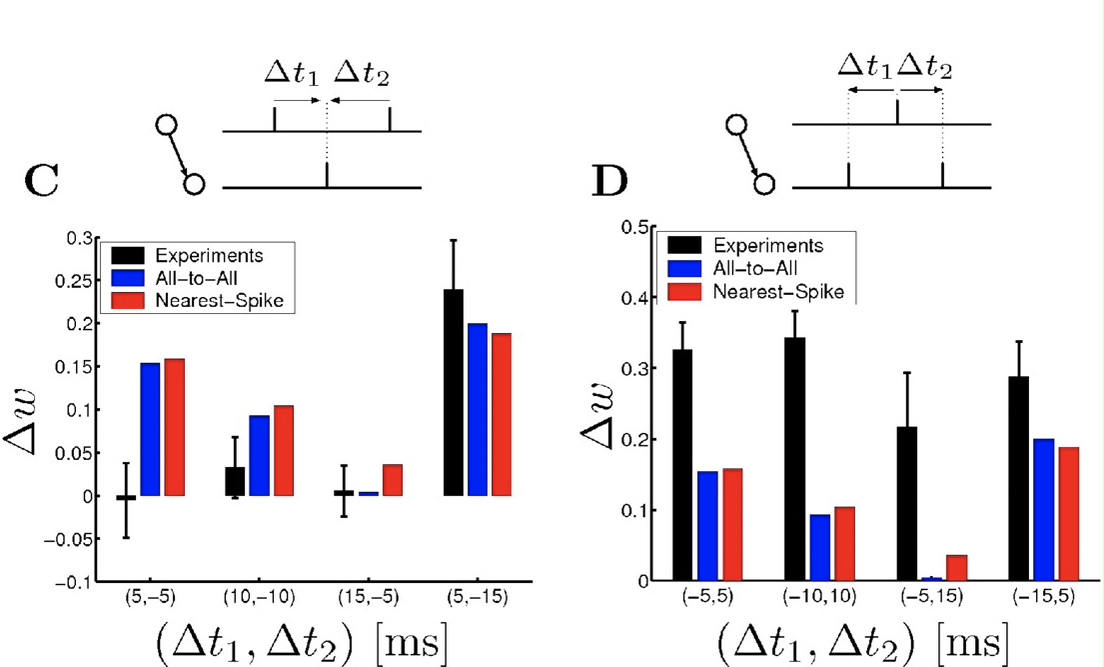### Triplet model with triplet protocol

Let us try to formulate the two triplet protocols using the triplet model and check if we can reproduce the asymmetry in the change in weights.

:

# Simulation
def run_triplet_protocol_simulation(neuron_model_name, synapse_model_name,
neuron_opts, nest_syn_opts,
spike_delays, n_triplets = 1, triplet_delay = 1000,
pre_post_pre=True):
dw_vec= []

# For pre-post-pre triplets
for _delays in spike_delays:
pre_spike_times, post_spike_times = create_triplet_spikes(_delays, n_triplets, triplet_delay)
if not pre_post_pre: # swap the spike arrays
post_spike_times, pre_spike_times = pre_spike_times, post_spike_times

sim_time = max(np.amax(pre_spike_times), np.amax(post_spike_times)) + 10. + 3 * syn_opts["delay"]

print('Simulating for (delta_t1, delta_t2) = ({}, {})'.format(_delays, _delays))
dw = run_triplet_stdp_network(neuron_model_name, synapse_model_name,
neuron_opts, nest_syn_opts,
pre_spike_times=pre_spike_times,
post_spike_times=post_spike_times,
sim_time=sim_time)
dw_vec.append(dw)

return dw_vec

:

# All to all - Parameters are taken from 
syn_opts = {
'delay': 1.,
'tau_minus': 33.7,
'tau_plus': 16.8,
'tau_x': 946.,
'tau_y': 27.,
'A2_plus': 6.1e-3,
'A3_plus': 6.7e-3,
'A2_minus': 1.6e-3,
'A3_minus': 1.4e-3,
'Wmax':  50.,
'Wmin' : 0.,
'w': 1.
}

synapse_suffix = neuron_model_name[neuron_model_name.find("_with_")+6:]
neuron_opts_nn = {'tau_minus__for_' + synapse_suffix: syn_opts['tau_minus'],
'tau_y__for_' + synapse_suffix: syn_opts['tau_y']}

nest_syn_opts = syn_opts.copy()
nest_syn_opts.pop('tau_minus')
nest_syn_opts.pop('tau_y')

:

27.0

:

# Nearest spike - Parameters are taken from 
syn_opts_nn = {
'delay': 1.,
'tau_minus': 33.7,
'tau_plus': 16.8,
'tau_x': 575.,
'tau_y': 47.,
'A2_plus': 4.6e-3,
'A3_plus': 9.1e-3,
'A2_minus': 3e-3,
'A3_minus': 7.5e-9,
'Wmax':  50.,
'Wmin' : 0.,
'w': 1.
}

synapse_suffix_nn = neuron_model_name_nn[neuron_model_name_nn.find("_with_")+6:]
neuron_opts_nn = {'tau_minus__for_' + synapse_suffix_nn: syn_opts_nn['tau_minus'],
'tau_y__for_' + synapse_suffix_nn: syn_opts_nn['tau_y']}

nest_syn_opts_nn = syn_opts_nn.copy()
nest_syn_opts_nn.pop('tau_minus')
nest_syn_opts_nn.pop('tau_y')

:

47.0


### pre-post-pre triplet

:

pre_post_pre_delays = [(5, -5), (10, -10), (15, -5), (5, -15)]

# All-to-All interation
dw_vec = run_triplet_protocol_simulation(neuron_model_name, synapse_model_name,
neuron_opts, nest_syn_opts,
pre_post_pre_delays,
n_triplets=1,
triplet_delay=1000)

# Nearest spike interaction
dw_vec_nn = run_triplet_protocol_simulation(neuron_model_name_nn, synapse_model_name_nn,
neuron_opts_nn, nest_syn_opts_nn,
pre_post_pre_delays,
n_triplets=1,
triplet_delay=1000)

Simulating for (delta_t1, delta_t2) = (5, -5)
Initial weight: 1.0, Updated weight: 1.0003735276417982
Simulating for (delta_t1, delta_t2) = (10, -10)
Initial weight: 1.0, Updated weight: 0.9998230228609227
Simulating for (delta_t1, delta_t2) = (15, -5)
Initial weight: 1.0, Updated weight: 0.9984719712644969
Simulating for (delta_t1, delta_t2) = (5, -15)
Initial weight: 1.0, Updated weight: 1.001383086591746
Simulating for (delta_t1, delta_t2) = (5, -5)
Initial weight: 1.0, Updated weight: 1.0005542494412774
Simulating for (delta_t1, delta_t2) = (10, -10)
Initial weight: 1.0, Updated weight: 1.0000931206450185
Simulating for (delta_t1, delta_t2) = (15, -5)
Initial weight: 1.0, Updated weight: 0.9991105337807658
Simulating for (delta_t1, delta_t2) = (5, -15)
Initial weight: 1.0, Updated weight: 1.0012383200640604

:

# Plot
bar_width = 0.25
fig, axes = plt.subplots()
br1 = np.arange(len(pre_post_pre_delays))
br2 = [x + bar_width for x in br1]
axes.bar(br1, dw_vec, width=bar_width, color='b')
axes.bar(br2, dw_vec_nn, width=bar_width, color='r')
axes.set_xticks([r + bar_width for r in range(len(br1))])
axes.set_xticklabels(pre_post_pre_delays)
axes.set_xlabel(r'${(\Delta{t_1}, \Delta{t_2})} \: [ms]$')
axes.set_ylabel(r'${\Delta}w$')
plt.show()### post-pre-post triplet

:

post_pre_post_delays = [(-5, 5), (-10, 10), (-5, 15), (-15, 5)]

# All-to-All interaction
dw_vec = run_triplet_protocol_simulation(neuron_model_name, synapse_model_name,
neuron_opts, nest_syn_opts,
post_pre_post_delays,
n_triplets=10,
triplet_delay=1000,
pre_post_pre=False)

# Nearest spike interaction
dw_vec_nn = run_triplet_protocol_simulation(neuron_model_name_nn, synapse_model_name_nn,
neuron_opts_nn, nest_syn_opts_nn,
post_pre_post_delays,
n_triplets=10,
triplet_delay=1000,
pre_post_pre=False)

Simulating for (delta_t1, delta_t2) = (-5, 5)
Initial weight: 1.0, Updated weight: 1.0452168105331474
Simulating for (delta_t1, delta_t2) = (-10, 10)
Initial weight: 1.0, Updated weight: 1.0275785817728278
Simulating for (delta_t1, delta_t2) = (-5, 15)
Initial weight: 1.0, Updated weight: 1.008936270857372
Simulating for (delta_t1, delta_t2) = (-15, 5)
Initial weight: 1.0, Updated weight: 1.050539844879153
Simulating for (delta_t1, delta_t2) = (-5, 5)
Initial weight: 1.0, Updated weight: 1.048644757755009
Simulating for (delta_t1, delta_t2) = (-10, 10)
Initial weight: 1.0, Updated weight: 1.026345906763637
Simulating for (delta_t1, delta_t2) = (-5, 15)
Initial weight: 1.0, Updated weight: 1.0099778920748412
Simulating for (delta_t1, delta_t2) = (-15, 5)
Initial weight: 1.0, Updated weight: 1.0466078732990223

:

# Plot
bar_width = 0.25
fig, axes = plt.subplots()
br1 = np.arange(len(pre_post_pre_delays))
br2 = [x + bar_width for x in br1]
axes.bar(br1, dw_vec, width=bar_width, color='b')
axes.bar(br2, dw_vec_nn, width=bar_width, color='r')
axes.set_xticks([r + bar_width for r in range(len(br1))])
axes.set_xticklabels(post_pre_post_delays)
axes.set_xlabel(r'${(\Delta{t_1}, \Delta{t_2})} \: [ms]$')
axes.set_ylabel(r'${\Delta}w$')
plt.show()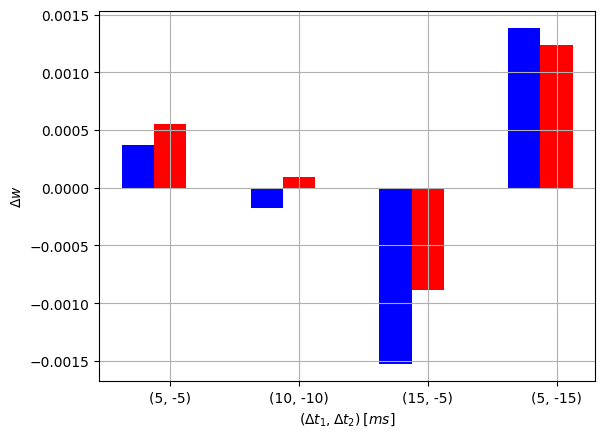This protocol is with a set of four spikes and defined as follows: a post-pre pair with a delay of $$\Delta{t_1} = t_1^{post} - t_1^{pre}< 0$$ is followed after a time $$T$$ with a pre-post pair with a delat of $$\Delta{t_2} = t_2^{post} - t_2^{pre} > 0$$. When $$T$$ is negative, a pre-post pair with a delay of $$\Delta{t_2} = t_2^{post} - t_2^{pre} > 0$$ is followed by a post-pre pair with delay $$\Delta{t_1} = t_1^{post} - t_1^{pre}< 0$$.

Try to formulate this protocol using the defined model and reproduce the following weight change window graph.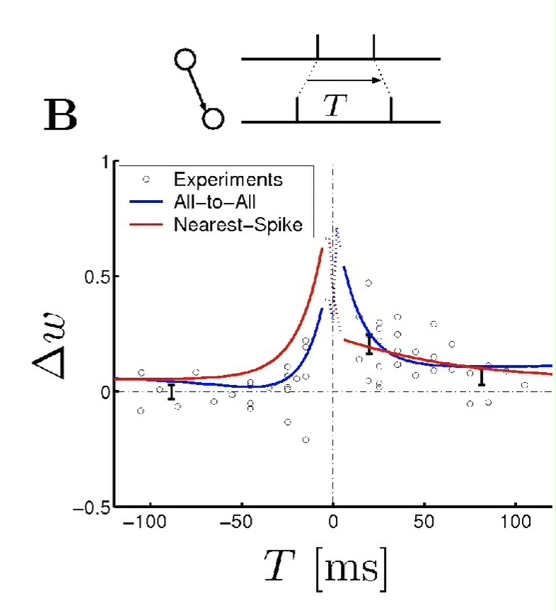## Acknowledgements

This software was developed in part or in whole in the Human Brain Project, funded from the European Union’s Horizon 2020 Framework Programme for Research and Innovation under Specific Grant Agreements No. 720270 and No. 785907 (Human Brain Project SGA1 and SGA2).